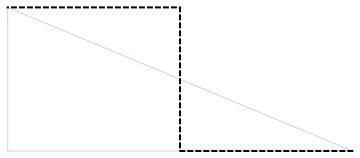# A Person Travels 6km Towards West, Then Travels 5km Towards North ,then Finally Travels 6km Towards West. Where Is He With Respect To His Starting Position?

The person is 12 km west and 5 km north of the starting position.
That is 13 km west-northwest of the start.

The distance is computed using the Pythagorean theorem. We recognize the triangle as a 5-12-13 triangle, so we can use that knowledge to write the answer without recomputing it.thanked the writer.

I'm familiar with the 3 x 4 x 5 right triangle, but I have never come across the 5-12-13 triangle to be able to recognize it as a 90 degree triangle.

So I started by using Oddman's graph and realizing that the displacement is 2*(2.5^w + 6^2)^1/2 or 13km.

Then, by using the tangent, the angle of the displacement from the origin (the east-west line) is approximately 22 degrees.

West-northwest is defined as:

The
direction or point on the mariner’s compass halfway between due west and
northeast, or 67 degrees, 30 minutes west of due north.

The distance between north and west is 90 degrees.  90-67 = 23, so that verifies the compass heading to be "west-northwest" of the starting point.

thanked the writer.